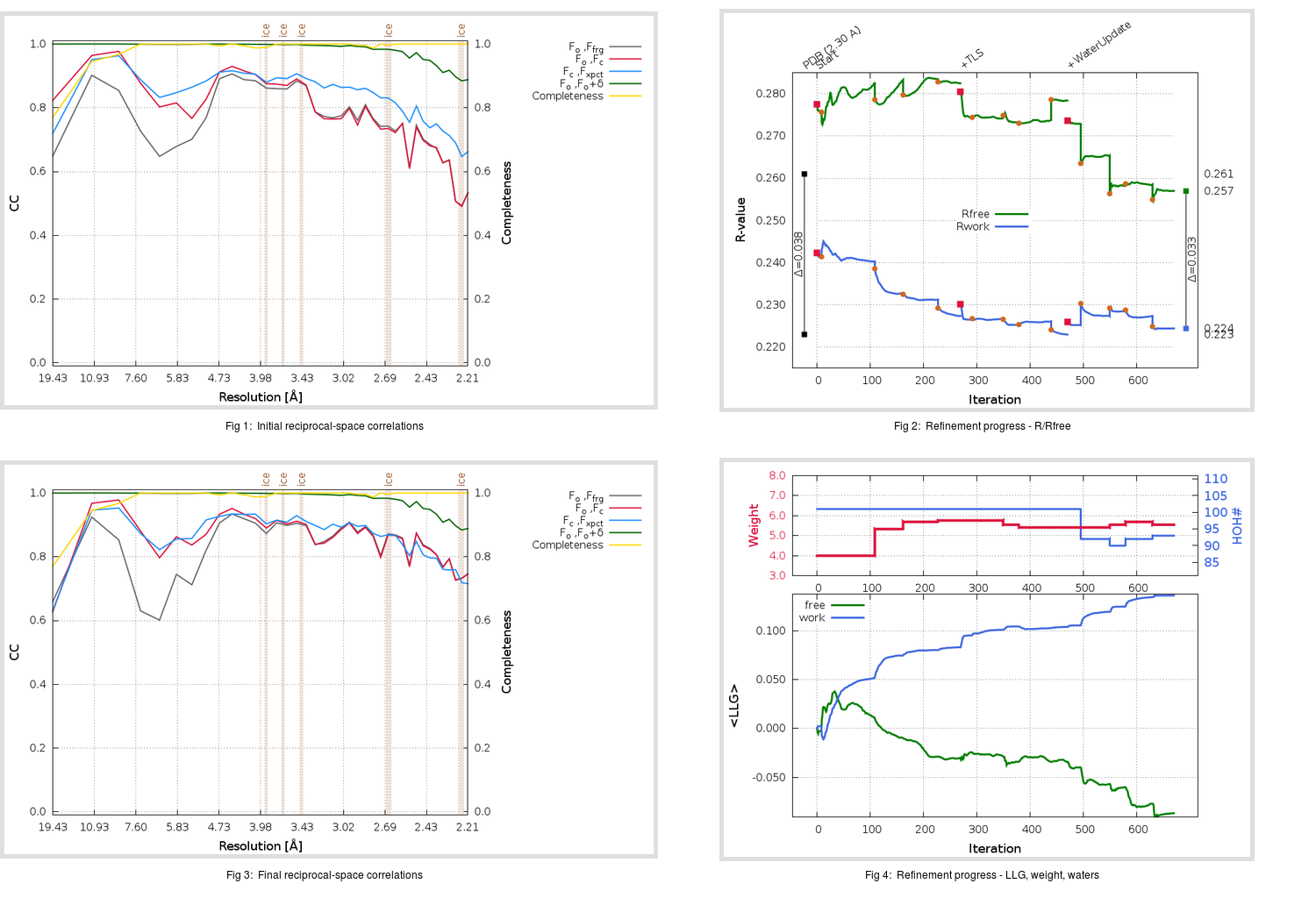Content:

```    Diffraction limits & principal axes of ellipsoid fitted to diffraction cut-off surface:
2.133         1.0000   0.0000   0.0000       0.894 a* - 0.447 b*
2.133         0.0000   1.0000   0.0000       b*
2.164         0.0000   0.0000   1.0000       c*
```

## Deposited

` `
 Date deposited Date data collection Resolution R, Rfree 20131110 20130402 2.30 0.2200 0.2610

Molprobity (CCP4 7.0 version) summary:

```Ramachandran outliers =   0.00 %
favored = 100.00 %
Rotamer outliers      =   1.83 %
C-beta deviations     =     0
Clashscore            =   0.50
RMS(bonds)            =   0.0028
RMS(angles)           =   0.58
MolProbity score      =   0.87
Resolution            =   2.30
R-work                =   0.2200
R-free                =   0.2610
```

```Number of waters      =   101

<B> (all atoms) =   42.51 ( sd =   17.59 ) for       1091 non-hydrogen atoms
<B>   (protein) =   42.20 ( sd =   17.93 ) for        980 non-hydrogen atoms
<B>     (water) =   42.13 ( sd =   10.12 ) for        101 non-hydrogen atoms
<B>    (others) =   76.40 ( sd =    7.82 ) for         10 non-hydrogen atoms

B min/max       (all non-hydrogen atoms) =   16.23 /  123.64
B min/max   (protein non-hydrogen atoms) =   16.23 /  123.64
B min/max     (water non-hydrogen atoms) =   16.55 /   69.91
B min/max     (other non-hydrogen atoms) =   65.33 /   87.74
```

## BUSTER (re-)refinement

` `

Molprobity (CCP4 7.0 version) summary:

```Ramachandran outliers =   0.00 %
favored =  99.21 %
Rotamer outliers      =   2.75 %
C-beta deviations     =     0
Clashscore            =   1.00
RMS(bonds)            =   0.0137
RMS(angles)           =   1.32
MolProbity score      =   1.13
Resolution            =   2.20
R-work                =   0.2243
R-free                =   0.2569
```

```Number of waters      =    93

<B> (all atoms) =   47.25 ( sd =   12.12 ) for       1083 non-hydrogen atoms
<B>   (protein) =   46.38 ( sd =   11.71 ) for        980 non-hydrogen atoms
<B>     (water) =   54.07 ( sd =   12.61 ) for         93 non-hydrogen atoms
<B>    (others) =   70.07 ( sd =    0.86 ) for         10 non-hydrogen atoms

B min/max       (all non-hydrogen atoms) =   23.05 /  111.83
B min/max   (protein non-hydrogen atoms) =   29.42 /   95.83
B min/max     (water non-hydrogen atoms) =   23.05 /  111.83
B min/max     (other non-hydrogen atoms) =   69.31 /   71.78
```

Refinement progression:Results:

` `
 File Remark 4NJL_aB_refine.01_03_refine.pdb.gz exact refinement commands are in header 4NJL_aB_refine.01_03_refine.mtz.gz including original deposited data and several re-refinement map coefficients 4NJL_aB_refine.01_03_BUSTER_model.cif.gz including any non-standard compound restraints 4NJL_aB_refine.01_03_BUSTER_refln.cif.gz